### Home > MC2 > Chapter 5 > Lesson 5.1.3 > Problem5-36

5-36.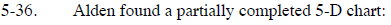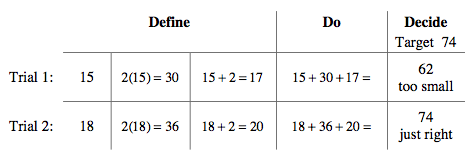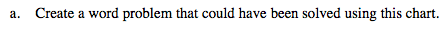Examine the chart in the Define and Do sections to figure out what the expression from the 5-D chart is, and the relationship between each of the units.

If the first unit is the variable, x, and the three units are addedtogether for the target value, 74, think of any possible wordproblems that could illustrate this 5-D chart.

The farthest house is twice as far as the closest house. The middle house is two more miles farther than the closest house. If the total distance is 74 miles, how far is the closest house?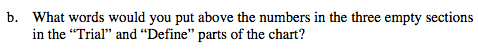Based on the expression and the word problem you created, which sections are the shortest, medium, or longest distance?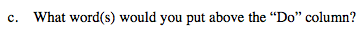Based on the word problem you created, what would this total represent?

From the example answer, the ''Do'' column could be the total distance for all three houses.